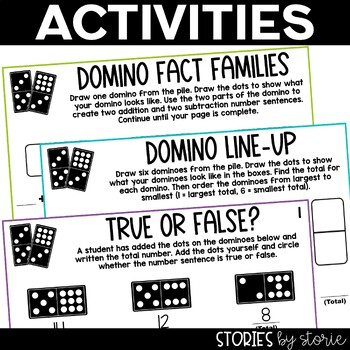# Dominoes Activities Distance LearningGrade Levels
1st - 2nd
Subjects
Standards
Resource Type
Formats Included
• PDF
•Google Apps™
Pages
42 pagesIncludes Google Apps™
The Teacher-Author indicated this resource includes assets from Google Workspace (e.g. docs, slides, etc.).

### Description

These domino activities include independent math games that students can play during centers and math rotations. They would be great for fast finishers, too! Grab a set of dominoes, print your pages, and have fun.

By placing these pages in sheet protectors or dry erase pockets, these domino activities can be reused many times with different results.

This resource also includes a Google Slides link. Once you make a copy and assign the activities to your students, they can drag and drop dominoes or colored dots into place and then complete the math skill.

Dominoes Activities

Domino Addition

Students will find the total number of dots on a domino. This includes options for both vertical and horizontal problems.

Domino Subtraction

Students will find the difference between the number of dots on both sides of the domino. This includes options for both vertical and horizontal problems.

Odds & Evens

Students will find the total number of dots on a domino. Then students must determine whether this is an odd or even number.

Domino Comparison

Students will draw two dominoes and find the total number of dots on each domino. Then students will compare the two totals using >, <, or =.

Domino Families

Students will draw a domino and use the two parts of the domino to create a fact family. They will record two addition and two subtraction problems that can be made using the numbers in the fact family.

Domino Line-Up

Students will draw six dominoes and find the total number of dots on each domino. Then students will rank the dominoes in order from largest to smallest.

True or False?

There are four student worksheets. For each, students look closely at one student's work and determine whether the answers match the dominoes shown or not. They will circle whether each answer is true or false. Answers are included for each worksheet.

What’s Missing?

There are four student worksheets. For each, students are shown one half of a domino and given a total sum. Students must determine the missing addend, or how many dots are needed to match the total shown below each domino. Answers are included for each worksheet.

Click on the preview to take a closer look at all of the domino activities included in this resource. You can also read more about this dominoes pack here.

•••••••••••••••••••••••••••••••••••••••••••••••••••••••••••••••••••••••••••••••••••••••••••••••••••••••••

You might also like:

Dice Games for Math Workshop

Addition and Subtraction Mazes

Math Games & Centers

•••••••••••••••••••••••••••••••••••••••••••••••••••••••••••••••••••••••••••••••••••••••••••••••••••••••••

Stay Connected with Stories by Storie

Blog

Instagram

Pinterest

Facebook

To find out about freebies, discounts, and new products, look for the ★ green star next to my store logo and click follow me.

If you have any questions, comments, or need changes made to this product to fit your classroom needs, please e-mail me: atozscrapbook@gmail.com. You can also contact me through my store's Q & A section.

© Stories by Storie - This item is intended for a SINGLE classroom. Duplication for other classrooms, an entire school, a school district, or for commercial purposes is strictly forbidden. Violations are subject to penalties of the Digital Millennium Copyright Act.

•••••••••••••••••••••••••••••••••••••••••••••••••••••••••••••••••••••••••••••••••••••••••••••••••••••••••

Total Pages
42 pages
Answer Key
Included
Teaching Duration
N/A
Report this Resource to TpT
Reported resources will be reviewed by our team. Report this resource to let us know if this resource violates TpT’s content guidelines.

### Standards

to see state-specific standards (only available in the US).
Determine whether a group of objects (up to 20) has an odd or even number of members, e.g., by pairing objects or counting them by 2s; write an equation to express an even number as a sum of two equal addends.
Fluently add and subtract within 20 using mental strategies. By end of Grade 2, know from memory all sums of two one-digit numbers.
Determine the unknown whole number in an addition or subtraction equation relating three whole numbers. For example, determine the unknown number that makes the equation true in each of the equations 8 + ? = 11, 5 = ▯ - 3, 6 + 6 = ▯.
Understand the meaning of the equal sign, and determine if equations involving addition and subtraction are true or false. For example, which of the following equations are true and which are false? 6 = 6, 7 = 8 - 1, 5 + 2 = 2 + 5, 4 + 1 = 5 + 2.
Add and subtract within 20, demonstrating fluency for addition and subtraction within 10. Use strategies such as counting on; making ten (e.g., 8 + 6 = 8 + 2 + 4 = 10 + 4 = 14); decomposing a number leading to a ten (e.g., 13 - 4 = 13 - 3 - 1 = 10 - 1 = 9); using the relationship between addition and subtraction (e.g., knowing that 8 + 4 = 12, one knows 12 - 8 = 4); and creating equivalent but easier or known sums (e.g., adding 6 + 7 by creating the known equivalent 6 + 6 + 1 = 12 + 1 = 13).

### Questions & Answers

Teachers Pay Teachers is an online marketplace where teachers buy and sell original educational materials.

More About Us